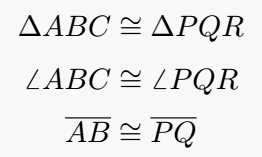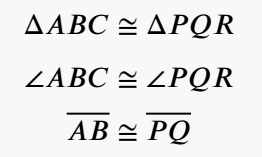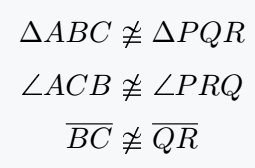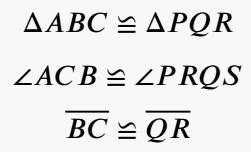# How do you type a Congruent(≅, ≇) symbol in LaTeX?

A congruent is a geometrical symbol formed by placing a tilde symbol on an equal symbol. One has more than one command and package.

And even if there is a difference in shape, you can understand which will be the best practice.

Symbol Congruent
Type of symbol Mathematics
Package No
Argument No
Latex command \cong
Example \cong → ≅
\documentclass{article}
\begin{document}
$\Delta ABC \cong \Delta PQR$
$\angle ABC \cong \angle PQR$
$\overline{AB} \cong \overline{PQ}$
\end{document}

Output :If all the properties of two triangles are equal, we use congruent symbol to show equivalent between them.

There are different packages like MnSymbol, fdsymbol, stix, etc. to complete the above task and each has the same command.

However, there are slight differences in the case of symbols. Default is the best practice.

\documentclass{article}
\usepackage{stix}
\begin{document}
$\Delta ABC \cong \Delta PQR$
$\angle ABC \cong \angle PQR$
$\overline{AB} \cong \overline{PQ}$
\end{document}

Output :## Not Congruent symbol in LaTeX

If all the properties of two triangles are not equal, we use not congruent symbol. Use of \ncong command is shown below.

\documentclass{article}
\usepackage{mathabx}
\begin{document}
$\Delta ABC \ncong \Delta PQR$
$\angle ACB \ncong \angle PRQS$
$\overline{BC} \ncong \overline{QR}$
\end{document}

Output :\ncong command is not default. However, the amsmath and mathabx packages have this command.

## Back Congruent symbol in LaTeX

In the case of the Back Congruent symbol, the tilde symbol is inverted on the equal symbol.

\documentclass{article}
\usepackage{stix}
\begin{document}
$\Delta ABC \backcong \Delta PQR$
$\angle ACB \backcong \angle PRQS$
$\overline{BC} \backcong \overline{QR}$
\end{document}

Output :#### Md Jidan Mondal

LaTeX expert with over 10 years of experience in document preparation and typesetting. Specializes in creating professional documents, reports, and presentations using LaTeX.